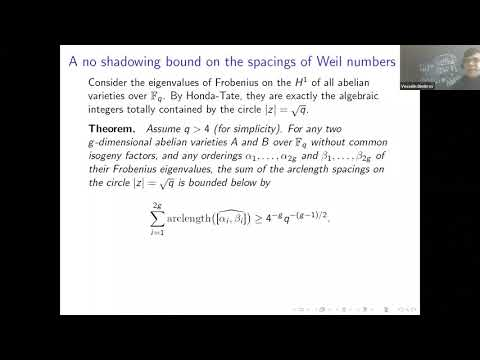## New constraints on the Galois configurations of algebraic integers in the complex plane

June 11, 2020
IAS
Presenters: Vesselin Dimitrov
Length: 1 hour

Watch Video

#### Abstract

Fekete (1923) discovered the notion of transfinite diameter while studying the possible configurations of Galois orbits of algebraic integers in the complex plane. Based purely on the fact that the discriminants of monic integer irreducible polynomials P(X) \in \mathbb{Z}[X] are at least $1$ in magnitude (since they are non-zero integers), he found that the incidences $(\mathcal{K}, P)$ between these polynomials $P(X)$ and compacts $\mathcal{K} \subset \mathbb{C}$ of transfinite diameter $d(\mathcal{K}) < 1$ have finite fibers over the argument $\mathcal{K}$. Here we say that $\mathcal{K}$ and $P$ are in incidence if all the roots of $P$ belong to $\mathcal{K}$. The descendants of Fekete's theorem are vast and powerful, notably including the equidistribution theorems of Bilu, Rumely and Szpiro-Ullmo-Zhang or -- in a different line of development -- the root separation bound of Mahler. But the input on the discriminant is sometimes too coarse to be useful: in reality one expects, but cannot prove, that discriminants of polynomials are large, and dropping them by integrality is too crude in certain finer questions such as Lehmer's.

Breusch (1951) solved the non-reciprocal case of the Lehmer problem by taking up a lossless arithmetic input from resultants rather than discriminants. In this talk, I will present some further lossless constraints that derive from certain whole infinite sequences of Hankel determinants attached to the polynomial $P(X)$ by algebraic operations. This will allow us to update on Fekete's theorem on the incidences $(\mathcal{K},P)$, by focusing this time on the fibers over the argument $P$ for compacts $\mathcal{K}$ that are made of finite unions of Jordan arcs continua covering the roots of $P$ with certain congruence conditions on $P$ and on the connected components of $\mathcal{K}$. The ensuing taming on Galois orbits turn out to be sufficiently severe to resolve the conjecture of Schinzel and Zassenhaus (I will explain this case in detail), amidst certain other cases of the Lehmer problem that are far off from Salem's extreme. In a geometric formulation for $\mathcal{A}_g$ with its Kobayashi metric, the root spacing constraints are likewise sufficiently severe to furthermore yield the exact $\mathcal{A}_g$ analogs of the well-known polynomial counting theorems of Penner and Leininger-Margalit on the "$L$-short" geodesics of moduli space $\mathcal{M}_g$.

Download Abstract